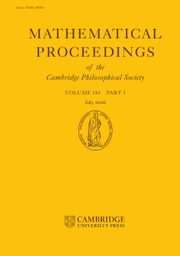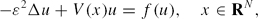HomeMathematical Proceedings of the Cambridge Philosophical Society

# Semi-classical bound states of Schrödinger equations

Published online by Cambridge University Press:  21 October 2013

Corresponding

## Abstract

We study the existence of semi-classical bound states of the nonlinear Schrödinger equation\begin{linenomath}$$-\varepsilon^2\Delta u+V(x)u=f(u),\quad x\in {\bf R}^N,$$\end{linenomath}
where N ≥ 3;, ϵ is a positive parameter; V:RN → [0, ∞) satisfies some suitable assumptions. We study two cases: if f is asymptotically linear, i.e., if lim|t| → ∞f(t)/t=constant, then we get positive solutions. If f is superlinear and f(u)=|u|p−2u+|u|q−2u, 2* > p > q > 2, we obtain the existence of multiple sign-changing semi-classical bound states with information on the estimates of the energies, the Morse indices and the number of nodal domains. For this purpose, we establish a mountain cliff theorem without the compactness condition and apply a new sign-changing critical point theorem.

Type
Research Article
Information

## Access options

Get access to the full version of this content by using one of the access options below.

## References

Ambrosetti, A., Badiale, M. and Cingolani, S.Semiclassical states of nonlinear Schrödinger equations. Arch. Ration. Mech. Anal. 140 (1997), 285300.CrossRefGoogle Scholar
Ambrosetti, A., Malchiodi, A. and Secchi, S.Multiplicity results for some nonlinear Schrödinger equations with potentials. Arch. Ration. Mech. Anal. 159 (2001), 253271.CrossRefGoogle Scholar
Ambrosotti, A. and Rabinowitz, P. H.Dual variational methods in critical point theory and applications. J. Func. Anal. 14 (1973), 349381.CrossRefGoogle Scholar
Bartsch, T.Critical point theory on partially ordered Hilbert spaces. J. Funct. Anal. 186 (2001), 117152.CrossRefGoogle Scholar
Bartsch, T., Liu, Z. and Weth, T.Sign changing solutions of superlinear Schrödinger equations. Comm. Partial Differential Equations 29 (2004), 2542.CrossRefGoogle Scholar
Brèzis, H. and Lieb, E.. A relation between pointwise convergence of functions and convergence of functionals. Proc. Amer. Math. Soc. 88 (1983), no. 3, 486490.CrossRefGoogle Scholar
Byeon, J. and Jeanjean, L.Standing waves for nonlinear Schrödinger equations with a general nonlinearity. Arch. Rational Mech. Anal. 185 (2007) 185200.CrossRefGoogle Scholar
Byeon, J. and Wang, Z. Q.Standing waves with a critical frequency for nonlinear Schrödinger equations II. Calc. Var. Partial Differential Equations 18 (2003), 207219.CrossRefGoogle Scholar
Conti, M., Merizzi, L. and Terracini, S.Remarks on variational methods and lower-upper solutions. NoDEA 6 (1999), 371393.CrossRefGoogle Scholar
Conti, M., Merizzi, L. and Terracini, S.On the existence of many solutions for a class of superlinear elliptic systems. J. Differential Equations 167 (2000), 357387.CrossRefGoogle Scholar
Dancer, N., Lam, K. Y. and Yan, S.The effect of the graph topology on the existence of multipeak solutions for nonlinear Schrödinger equations. Abstr. Appl. Anal. 3 (1998), 293318.CrossRefGoogle Scholar
Dancer, N. and Yan, S.On the existence of multipeak solutions for nonlinear field equations on$\mathbb{R}^N$. Discrete Contin. Dyn. Syst 6 (2000), 3950.Google Scholar
Dávila, J., del Pino, M., Musso, M. and Wei, J.. Standing waves for supercritical nonlinear Schrödinger equations. J. Differential Equations 236 (2007), 164198.CrossRefGoogle Scholar
Del Pino, M. and Felmer, P. L.. Local mountain passes for semilinear elliptic problems in unbounded domains. Calc. Var. Partial Differential Equations 4 (1996), 121137.CrossRefGoogle Scholar
Del Pino, M. and Felmer, P. L.. Semi-classical states for nonlinear Schrödinger equations. J. Funct. Anal. 149 (1997), 245265.CrossRefGoogle Scholar
Del Pino, M. and Felmer, P. L.. Semi-classical states for nonlinear Schrödinger equations: a variational reduction method. Math. Ann. 324 (2002), 132.CrossRefGoogle Scholar
Ding, Y. and Lin, F.Solutions of perturbed Schr?dinger equations with critical nonlinearity. Calc. Var. Partial Differential Equations 30 (2007), 231249.CrossRefGoogle Scholar
Floer, A. and Weinstein, A.Nonspreading wave packets for the cubic Schrödinger equation with a bounded potential. J. Funct. Anal. 69 (1986), 397408.CrossRefGoogle Scholar
Jeanjean, L. and Tanaka, K.Singularly perturbed elliptic problems with superlinear or asymptotically linear nonlinearities. Calc. Var. Partial Differential Equations 21 (2004), 287318.CrossRefGoogle Scholar
Kang, X. and Wei, J.On interacting bumps of semi-classical states of nonlinear Schrödinger equations. Adv. Differential Equations 5 (2000), 899928.Google Scholar
Jeanjean, L.On the existence of bounded Palais-Smale sequences and application to a Landesman–Lazer type problem set on RN. Proc. Roy. Soc. Edinburgh Sect. A 129 (1999), 787809.CrossRefGoogle Scholar
Jeanjean, L. Local conditions insuring bifurcation from the continuous spectrum. Math. Z. 232 (1999), 651664.Google Scholar
Jeanjean, L. Bounded Palais–Smale sequences in minimax theorems and applications to bifurcation theory. Variational problems and related topics (Japanese) (Kyoto, 2000). No. 1181 (2001), 8086.Google Scholar
Jeanjean, L. and Toland, J. F.Bounded Palais–Smale mountain-pass sequences. C. R. Acad. Sci. Paris Sr. I Math. 327 (1998), 2328.CrossRefGoogle Scholar
Kavian, O.Introduction à la Théorie des Points Critiques (Springer, New York, 1993).Google Scholar
Li, Y. Y.On a singularly perturbed elliptic equation. Adv. Differential Equations. 2 (1997), 955980.Google Scholar
Oh, Y.–G.. Existence of semiclassical bound states of nonlinear Schrödinger equations with potentials of the class (V)a. Comm. Partial Differential Equation 13 (1988), 14991519.CrossRefGoogle Scholar
Oh, Y.–G.. Stability of semiclassical bound states of nonlinear Schrödinger equations with potentials. Comm. Math. Phys. 121 (1989), 1133.CrossRefGoogle Scholar
Rabinowitz, P. H.On a class of nonlinear Schrödinger equations. Z. Angew. Math. Phys. 43 (1992), 270291.CrossRefGoogle Scholar
Rabinowitz, P. H. Multibump solutions of a semilinear elliptic PDE on\$\mathbb{R}^n, Degenerate diffusions (Minneapolis, MN, 1991), 175185, IMA Vol. Math. Appl. 47 (Springer, New York, 1993).Google Scholar
Rozenbljum, G. V.Distribution of the discrete spectrum of singular differential operator. Dokl. Akad. Nauk SSSR 202 (1972), 10121015; Soviet Math. Dokl. 13 (1972), 245–249.Google Scholar
Schechter, M.A variation of the mountain pass lemma and applications. J. London Math. Soc. 44 (2) (1991), 491502.CrossRefGoogle Scholar
Schecher, M. and Zou, W.Sign-changing critical points of linking type theorems. Trans. Amer. Math. Soc. 358 (2006), 52935318.CrossRefGoogle Scholar
Solimini, S.A note on compactness-type properties with respect to Lorenz norms of bounded subsets of a Sobolev spaces. Ann. Inst. H. Poincaré-Anal. Non Linéaire 12 (1995), 319337.CrossRefGoogle Scholar
Strauss, W. A.Existence of solitary waves in higher dimensions. Comm. Math. Phys. 55 (1977), 149162.CrossRefGoogle Scholar
Struwe, M.Variational Methods. Springer, Second Edition, 1996.Google Scholar
Wang, X.On concentration of positive bound states of nonlinear Schrödinger equations. Comm. Math. Phys. 153 (1993), 229244.CrossRefGoogle Scholar
Zou, W.Sign-Changing Critical Points Theory (Springer-New York, 2008).Google Scholar
Zou, W. Infinitely many nodal bound states for a Schrödinger equation. preprint.Google Scholar

### Full text views

Full text views reflects PDF downloads, PDFs sent to Google Drive, Dropbox and Kindle and HTML full text views.

Total number of HTML views: 0
Total number of PDF views: 36 *
View data table for this chart

* Views captured on Cambridge Core between September 2016 - 22nd January 2021. This data will be updated every 24 hours.

Hostname: page-component-76cb886bbf-86jzp Total loading time: 0.481 Render date: 2021-01-22T16:46:15.332Z Query parameters: { "hasAccess": "0", "openAccess": "0", "isLogged": "0", "lang": "en" } Feature Flags: { "shouldUseShareProductTool": true, "shouldUseHypothesis": true, "isUnsiloEnabled": true, "metricsAbstractViews": false, "figures": false, "newCiteModal": false }

# Send article to Kindle

Note you can select to send to either the @free.kindle.com or @kindle.com variations. ‘@free.kindle.com’ emails are free but can only be sent to your device when it is connected to wi-fi. ‘@kindle.com’ emails can be delivered even when you are not connected to wi-fi, but note that service fees apply.

Find out more about the Kindle Personal Document Service.

Semi-classical bound states of Schrödinger equations
Available formats
×

# Send article to Dropbox

To send this article to your Dropbox account, please select one or more formats and confirm that you agree to abide by our usage policies. If this is the first time you use this feature, you will be asked to authorise Cambridge Core to connect with your <service> account. Find out more about sending content to Dropbox.

Semi-classical bound states of Schrödinger equations
Available formats
×

# Send article to Google Drive

To send this article to your Google Drive account, please select one or more formats and confirm that you agree to abide by our usage policies. If this is the first time you use this feature, you will be asked to authorise Cambridge Core to connect with your <service> account. Find out more about sending content to Google Drive.

Semi-classical bound states of Schrödinger equations
Available formats
×
×